# SPARSE-MOD COVID-19 Model

#### July 2022

library(SPARSEMODr)
library(future.apply)
#> Warning: package 'future' was built under R version 4.1.2
library(tidyverse)
library(viridis)
library(lubridate)

# To run in parallel, use, e.g., plan("multisession"):
future::plan("sequential")

## COVID-19 model with time-varying hospitalization rates

Now we will demonstrate how the SPARSEMODr COVID-19 model can be used to understand dynamics when hospitalization rates change over time. This can happen, for instance, if the age-distribution of cases changes over time: if younger folks dominate transmission for a period of time, then we would expect fewer hospitalizations.

### Generating a synthetic meta-population

First, we will generate some data that describes the meta-population1 of interest.

# Set seed for reproducibility
set.seed(5)

# Number of focal populations:
n_pop = 100

# Population sizes + areas
## Draw from neg binom:
census_area = rnbinom(n_pop, mu = 50, size = 3)

# Identification variable for later
pop_ID = c(1:n_pop)

# Assign coordinates, plot for reference
lat_temp = runif(n_pop, 32, 37)
long_temp = runif(n_pop, -114, -109)

# Storage:
region = rep(NA, n_pop)
pop_N = rep(NA, n_pop)

# Assign region ID and population size
for(i in 1 : n_pop){
if ((lat_temp[i] >= 34.5) & (long_temp[i] <= -111.5)){
region[i] = "1"
pop_N[i] = rnbinom(1, mu = 50000, size = 2)
} else if((lat_temp[i] >= 34.5) & (long_temp[i] > -111.5)){
region[i] = "2"
pop_N[i] = rnbinom(1, mu = 10000, size = 3)
} else if((lat_temp[i] < 34.5) & (long_temp[i] > -111.5)){
region[i] = "4"
pop_N[i] = rnbinom(1, mu = 50000, size = 2)
} else if((lat_temp[i] < 34.5) & (long_temp[i] <= -111.5)){
region[i] = "3"
pop_N[i] = rnbinom(1, mu = 10000, size = 3)
}
}

pop_local_df =
data.frame(pop_ID = pop_ID,
pop_N = pop_N,
census_area,
lat = lat_temp,
long = long_temp,
region = region)

# Plot the map:
pop_plot = ggplot(pop_local_df) +
geom_point(aes(x = long, y = lat,
fill = region, size = pop_N),
shape = 21) +
scale_size(name = "Pop. Size", range = c(1,5),
breaks = c(5000, 50000, 150000)) +
scale_fill_manual(name = "Region", values = c("#00AFBB", "#D16103",
"#E69F00", "#4E84C4")) +
geom_hline(yintercept = 34.5, colour = "#999999", linetype = 2) +
geom_vline(xintercept = -111.5, colour = "#999999", linetype = 2) +
guides(size = guide_legend(order = 2),
fill = guide_legend(order = 1,
override.aes = list(size = 3))) +
# Map coord
coord_quickmap() +
theme_classic() +
theme(
axis.line = element_blank(),
axis.title = element_blank(),
plot.margin = unit(c(0, 0.1, 0, 0), "cm")
)

pop_plot

# Calculate pairwise dist
## in meters so divide by 1000 for km
dist_mat = geosphere::distm(cbind(pop_local_df$long, pop_local_df$lat))/1000
hist(dist_mat, xlab = "Distance (km)", main = "")

# We need to determine how many Exposed individuals
# are present at the start in each population
E_pops = vector("numeric", length = n_pop)
# We'll assume a total number of exposed across the
# full meta-community, and then randomly distribute these hosts
n_initial_E = 20
# (more exposed in larger populations)
these_E <- sample.int(n_pop,
size = n_initial_E,
replace = TRUE,
prob = pop_N)
for(i in 1:n_initial_E){
E_pops[these_E[i]] <- E_pops[these_E[i]] + 1
}

pop_local_df$E_pops = E_pops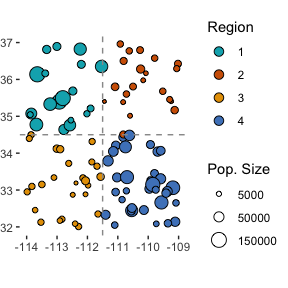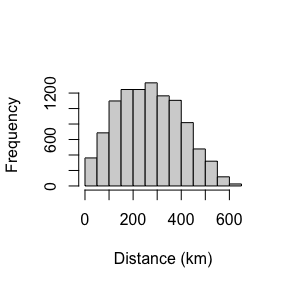### Setting up the time-windows One of the benefits of the SPARSEMODr design is that the user can specify how certain parameters of the model change over time (see our vignette on key features of SPARSEMODr for more details). In this particular example, we allow the time-varying transmission rate to change in a step-wise fashion, due for instance to changing behaviors in the host population. We further allow the hospitalization rates to change over time, again step-wise. Note that when the parameter values change between two time windows, the model assumes a linear change over the number of days in that window. In other words, the user specifies the value of the parameter achieved on the last day of the time window. Of course, the user could specify daily values for these parameter values to gain more control (see our vignette on the SPARSEMOD SEIR model for more details). We’ll use the $$\texttt{time_windows()}$$ function to generate patterns of time-varying R0 and hospitalization rates, but here we show how it works behind the scenes (manually): # Set up the dates of change. 5 time windows n_windows = 5 # Window intervals start_dates = c(mdy("1-1-20"), mdy("2-1-20"), mdy("2-16-20"), mdy("3-11-20"), mdy("3-22-20")) end_dates = c(mdy("1-31-20"), mdy("2-15-20"), mdy("3-10-20"), mdy("3-21-20"), mdy("5-1-20")) # Date sequence date_seq = seq.Date(start_dates, end_dates[n_windows], by = "1 day") # Time-varying beta and hospitalization rate # Note that these are not necessarily realistic hospitalization rates! changing_beta = c(0.3, 0.1, 0.1, 0.15, 0.15) changing_hr = c(0.5, 0.3, 0.3, 0.1, 0.1) #beta sequence beta_seq = NULL hr_seq = NULL beta_seq[1:(yday(end_dates) - yday(start_dates) + 1)] = changing_beta hr_seq[1:(yday(end_dates) - yday(start_dates) + 1)] = changing_hr for(i in 2:n_windows){ beta_temp_seq = NULL beta_temp = NULL hr_temp_seq = NULL hr_temp = NULL # beta time steps... if(changing_beta[i] != changing_beta[i-1]){ beta_diff = changing_beta[i-1] - changing_beta[i] n_days = yday(end_dates[i]) - yday(start_dates[i]) + 1 beta_slope = - beta_diff / n_days for(j in 1:n_days){ beta_temp_seq[j] = changing_beta[i-1] + beta_slope*j } }else{ n_days = yday(end_dates[i]) - yday(start_dates[i]) + 1 beta_temp_seq = rep(changing_beta[i], times = n_days) } beta_seq = c(beta_seq, beta_temp_seq) # Hosp rate time steps... if(changing_hr[i] != changing_hr[i-1]){ hr_diff = changing_hr[i-1] - changing_hr[i] n_days = yday(end_dates[i]) - yday(start_dates[i]) + 1 hr_slope = - hr_diff / n_days for(j in 1:n_days){ hr_temp_seq[j] = changing_hr[i-1] + hr_slope*j } }else{ n_days = yday(end_dates[i]) - yday(start_dates[i]) + 1 hr_temp_seq = rep(changing_hr[i], times = n_days) } hr_seq = c(hr_seq, hr_temp_seq) } beta_seq_df = data.frame(beta_seq, date_seq) date_breaks = seq(range(date_seq), range(date_seq), by = "1 month") ggplot(beta_seq_df) + geom_path(aes(x = date_seq, y = beta_seq)) + scale_x_date(breaks = date_breaks, date_labels = "%b") + labs(x="", y=expression("Time-varying "*beta*", ("*beta[t]*")")) + # THEME theme_classic()+ theme( axis.text = element_text(size = 10, color = "black"), axis.title = element_text(size = 12, color = "black"), axis.text.x = element_text(angle = 45, vjust = 0.5) ) hr_seq_df = data.frame(hr_seq, date_seq) date_breaks = seq(range(date_seq), range(date_seq), by = "1 month") ggplot(hr_seq_df) + geom_path(aes(x = date_seq, y = hr_seq)) + scale_x_date(breaks = date_breaks, date_labels = "%b") + labs(x="", y="Time-varying Hosp. Rate") + # THEME theme_classic()+ theme( axis.text = element_text(size = 10, color = "black"), axis.title = element_text(size = 12, color = "black"), axis.text.x = element_text(angle = 45, vjust = 0.5) )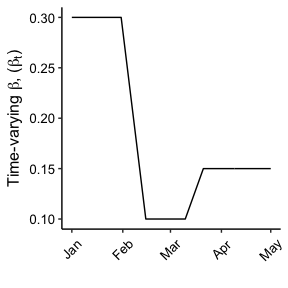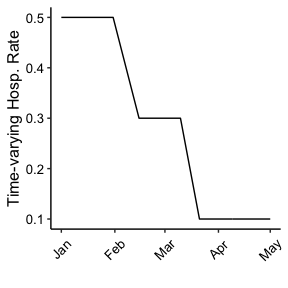# Set up the dates of change. 5 time windows ### TIME-VARYING PARAMETERS ### ### Now need a daily sequence ### n_days = length(beta_seq) # Migration rate changing_m = rep(1/10.0, times = n_days) # Migration range changing_dist_phi = rep(150, times = n_days) # Immigration (none) changing_imm_frac = rep(0, times = n_days) # Date Sequence for later: date_seq = seq.Date(start_dates, end_dates[n_windows], by = "1 day") # Create the time_window() object tw = time_windows( beta = beta_seq, m = changing_m, dist_phi = changing_dist_phi, imm_frac = changing_imm_frac, hosp_rate = hr_seq, daily = date_seq ) # Create the covid19_control() object covid19_control <- covid19_control(input_N_pops = pop_N, input_E_pops = E_pops) #> Parameter input_I_asym_pops was not specified; assuming to be zeroes. #> Parameter input_I_presym_pops was not specified; assuming to be zeroes. #> Parameter input_I_sym_pops was not specified; assuming to be zeroes. #> Parameter input_I_home_pops was not specified; assuming to be zeroes. #> Parameter input_I_hosp_pops was not specified; assuming to be zeroes. #> Parameter input_I_icu1_pops was not specified; assuming to be zeroes. #> Parameter input_I_icu2_pops was not specified; assuming to be zeroes. #> Parameter input_R_pops was not specified; assuming to be zeroes. #> Parameter input_D_pops was not specified; assuming to be zeroes. ### Running the COVID-19 model in parallel Now we have all of the input elements needed to run SPARSEMODr’s COVID-19 model. Below we demonstrate a workflow to generate stochastic realizations of the model in parallel. # How many realizations of the model? n_realz = 75 # Need to assign a distinct seed for each realization ## Allows for reproducibility input_realz_seeds = c(1:n_realz) # Run the model in parallel model_output = model_parallel( # Necessary inputs input_dist_mat = dist_mat, input_census_area = pop_local_df$census_area,
input_tw = tw,
input_realz_seeds = input_realz_seeds,
control = covid19_control,
# OTHER MODEL PARAMS
trans_type = 1, # freq-dependent trans
stoch_sd = 2.0  # stoch transmission sd
)

glimpse(model_output)
#> Rows: 915,000
#> Columns: 19
#> $pops.seed <int> 1, 1, 1, 1, 1, 1, 1, 1, 1, 1, 1, 1, 1, 1, 1, 1, 1, 1… #>$ pops.pop          <int> 1, 2, 3, 4, 5, 6, 7, 8, 9, 10, 11, 12, 13, 14, 15, 1…
#> $pops.time <int> 1, 1, 1, 1, 1, 1, 1, 1, 1, 1, 1, 1, 1, 1, 1, 1, 1, 1… #>$ pops.S_pop        <int> 118910, 8765, 10066, 86540, 11709, 14078, 10111, 538…
#> $pops.E_pop <int> 1, 0, 0, 2, 1, 0, 0, 0, 0, 0, 0, 0, 1, 1, 0, 0, 0, 0… #>$ pops.I_asym_pop   <int> 0, 0, 0, 0, 0, 0, 0, 0, 0, 0, 0, 0, 0, 0, 0, 0, 0, 0…
#> $pops.I_presym_pop <int> 0, 0, 0, 0, 0, 0, 0, 0, 0, 0, 0, 0, 0, 0, 0, 0, 0, 0… #>$ pops.I_sym_pop    <int> 0, 0, 0, 0, 0, 0, 0, 0, 0, 0, 0, 0, 0, 0, 0, 0, 0, 0…
#> $pops.I_home_pop <int> 0, 0, 0, 0, 0, 0, 0, 0, 0, 0, 0, 0, 0, 0, 0, 0, 0, 0… #>$ pops.I_hosp_pop   <int> 0, 0, 0, 0, 0, 0, 0, 0, 0, 0, 0, 0, 0, 0, 0, 0, 0, 0…
#> $pops.I_icu1_pop <int> 0, 0, 0, 0, 0, 0, 0, 0, 0, 0, 0, 0, 0, 0, 0, 0, 0, 0… #>$ pops.I_icu2_pop   <int> 0, 0, 0, 0, 0, 0, 0, 0, 0, 0, 0, 0, 0, 0, 0, 0, 0, 0…
#> $pops.R_pop <int> 0, 0, 0, 0, 0, 0, 0, 0, 0, 0, 0, 0, 0, 0, 0, 0, 0, 0… #>$ pops.D_pop        <int> 0, 0, 0, 0, 0, 0, 0, 0, 0, 0, 0, 0, 0, 0, 0, 0, 0, 0…
#> $events.pos <int> 1, 0, 0, 2, 0, 0, 0, 0, 0, 0, 0, 0, 0, 0, 0, 0, 0, 0… #>$ events.sym        <int> 0, 0, 0, 0, 0, 0, 0, 0, 0, 0, 0, 0, 0, 0, 0, 0, 0, 0…
#> $events.total_hosp <int> 0, 0, 0, 0, 0, 0, 0, 0, 0, 0, 0, 0, 0, 0, 0, 0, 0, 0… #>$ events.total_icu  <int> 0, 0, 0, 0, 0, 0, 0, 0, 0, 0, 0, 0, 0, 0, 0, 0, 0, 0…
#> $events.n_death <int> 0, 0, 0, 0, 0, 0, 0, 0, 0, 0, 0, 0, 0, 0, 0, 0, 0, 0… ### Plotting the output First we need to manipulate and aggregate the output data. Here we show an example just using the ‘new events’ that occur each day. # Grab the new events variables new_events_df = model_output %>% select(pops.seed:pops.time, events.pos:events.n_death) # Simplify/clarify colnames colnames(new_events_df) = c("iter","pop_ID","time", "new_pos", "new_sym", "new_hosp", "new_icu", "new_death") # Join the region region_df = pop_local_df %>% select(pop_ID, region) new_events_df = left_join(new_events_df, region_df, by = "pop_ID") # Join with dates (instead of "time" integer) date_df = data.frame( date = date_seq, time = c(1:length(date_seq)) ) new_events_df = left_join(new_events_df, date_df, by = "time") # Aggregate outcomes by region: ## First, get the sum across regions,dates,iterations new_event_sum_df = new_events_df %>% group_by(region, iter, date) %>% summarize(new_pos = sum(new_pos), new_sym = sum(new_sym), new_hosp = sum(new_hosp), new_icu = sum(new_icu), new_death = sum(new_death)) #> summarise() has grouped output by 'region', 'iter'. You can override using the .groups argument. glimpse(new_event_sum_df) #> Rows: 36,600 #> Columns: 8 #> Groups: region, iter  #>$ region    <chr> "1", "1", "1", "1", "1", "1", "1", "1", "1", "1", "1", "1", …
#> $iter <int> 1, 1, 1, 1, 1, 1, 1, 1, 1, 1, 1, 1, 1, 1, 1, 1, 1, 1, 1, 1, … #>$ date      <date> 2020-01-01, 2020-01-02, 2020-01-03, 2020-01-04, 2020-01-05,…
#> $new_pos <int> 1, 0, 2, 1, 1, 3, 4, 1, 1, 2, 6, 5, 4, 3, 8, 6, 6, 14, 12, 6… #>$ new_sym   <int> 0, 0, 0, 0, 0, 0, 1, 1, 1, 1, 3, 2, 2, 2, 7, 0, 6, 5, 9, 9, …
#> $new_hosp <int> 0, 0, 0, 0, 0, 0, 0, 0, 0, 0, 0, 0, 0, 1, 2, 1, 0, 1, 3, 2, … #>$ new_icu   <int> 0, 0, 0, 0, 0, 0, 0, 0, 0, 0, 0, 0, 0, 0, 0, 1, 0, 1, 0, 0, …
#> $new_death <int> 0, 0, 0, 0, 0, 0, 0, 0, 0, 0, 0, 0, 0, 0, 0, 0, 0, 1, 1, 0, … # Now calculate the median model trajectory across the realizations new_event_median_df = new_event_sum_df %>% ungroup() %>% group_by(region, date) %>% summarize(med_new_pos = median(new_pos), med_new_sym = median(new_sym), med_new_hosp = median(new_hosp), med_new_icu = median(new_icu), med_new_death = median(new_death)) #> summarise() has grouped output by 'region'. You can override using the .groups argument. glimpse(new_event_median_df) #> Rows: 488 #> Columns: 7 #> Groups: region  #>$ region        <chr> "1", "1", "1", "1", "1", "1", "1", "1", "1", "1", "1", "…
#> $date <date> 2020-01-01, 2020-01-02, 2020-01-03, 2020-01-04, 2020-01… #>$ med_new_pos   <int> 2, 1, 1, 1, 1, 1, 1, 2, 2, 2, 3, 3, 4, 3, 6, 5, 6, 7, 8,…
#> $med_new_sym <int> 0, 0, 0, 0, 0, 0, 0, 1, 1, 1, 1, 1, 2, 2, 2, 2, 3, 3, 4,… #>$ med_new_hosp  <int> 0, 0, 0, 0, 0, 0, 0, 0, 0, 0, 0, 0, 0, 0, 0, 0, 0, 1, 1,…
#> $med_new_icu <int> 0, 0, 0, 0, 0, 0, 0, 0, 0, 0, 0, 0, 0, 0, 0, 0, 0, 0, 0,… #>$ med_new_death <int> 0, 0, 0, 0, 0, 0, 0, 0, 0, 0, 0, 0, 0, 0, 0, 0, 0, 0, 0,…

Now we’ll start creating a rather complex figure to show the different time intervals. We’ll layer on the elements. For this example, we’ll just look at the number of new hospitalizations per region.

# SET UP SOME THEMATIC ELEMENTS:
## Maximum value of the stoch trajectories, for y axis ranges
max_hosp = max(new_event_sum_df$new_hosp) ## Breaks for dates: date_breaks = seq(range(date_seq), range(date_seq), by = "1 month") ####################### # PLOT ####################### # First we'll create an element list for plotting: plot_new_hosp_base = list( # Date Range: scale_x_date(limits = range(date_seq), breaks = date_breaks, date_labels = "%b"), # New Hosp Range: scale_y_continuous(limits = c(0, max_hosp*1.05)), # BOXES AND TEXT TO LABEL TIME WINDOWS ## beta = 3.0 annotate("text", label = paste0("beta = ", format(changing_beta, nsmall = 1)), color = "#39558CFF", hjust = 0, vjust = 1, size = 3.0, x = start_dates, y = max_hosp*1.05), annotate("rect", xmin = start_dates, xmax = end_dates, ymin = 0, ymax = max_hosp*1.05, fill = "gray", alpha = 0.2), ## beta = 0.8 annotate("text", label = paste0("beta = ", changing_beta), color = "#39558CFF", hjust = 0, vjust = 1, size = 3.0, x = start_dates, y = max_hosp*1.05), annotate("rect", xmin = start_dates, xmax = end_dates, ymin = 0, ymax = max_hosp*1.05, fill = "gray", alpha = 0.2), ## beta = 1.4 annotate("text", label = paste0("beta = ", changing_beta), color = "#39558CFF", hjust = 0, vjust = 1, size = 3.0, x = start_dates, y = max_hosp*1.05), annotate("rect", xmin = start_dates, xmax = end_dates, ymin = 0, ymax = max_hosp*1.05, fill = "gray", alpha = 0.2), # THEME ELEMENTS labs(x = "", y = "New Hospitalizations Per Day"), theme_classic(), theme( axis.text = element_text(size = 12, color = "black"), axis.title = element_text(size = 14, color = "black"), axis.text.x = element_text(angle = 45, vjust = 0.5) ) ) ggplot() + plot_new_hosp_base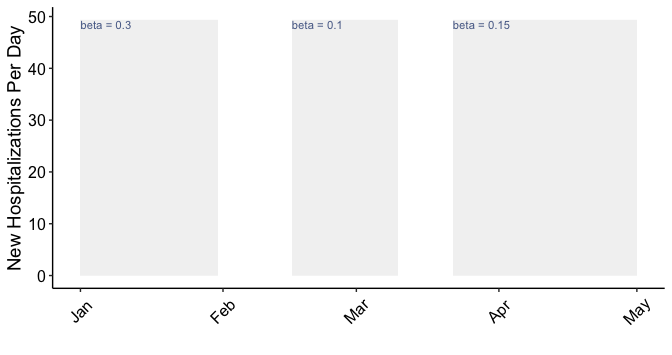Ok, now we have our plotting base, and we’ll layer on the model output. We’ll add the stochastic trajectories as well as the median model trajectory.  # region labels for facets: region_labs = paste0("Region ", sort(unique(region_df$region)))
names(region_labs) = sort(unique(region_df\$region))

# Create the plot:
plot_new_hosp =
ggplot() +
# Facet by Region
facet_wrap(~region,
scales = "free",
labeller = labeller(region = region_labs)) +
plot_new_hosp_base +
geom_path(data = new_event_sum_df,
aes(x = date, y = new_hosp, group = iter, color = region),
alpha = 0.05) +
geom_path(data = new_event_median_df,
aes(x = date, y = med_new_hosp, color = region),
size = 2) +
# Colors per region:
scale_color_manual(values = c("#00AFBB", "#D16103",
"#E69F00", "#4E84C4")) +
guides(color="none")

plot_new_hosp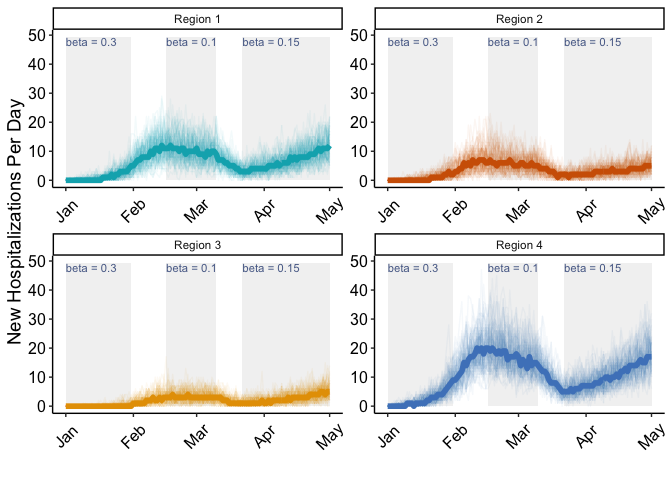1. A set of distinct, focal populations that are connected by migration↩︎